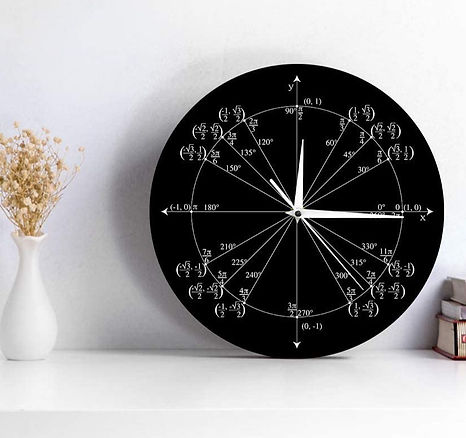top of page

# Unit Circle ClockThe Unit Circle Clock is an ingenious application of the unit circle known from trigonometry...

A unit circle clock is a type of clock that tells time using angles on a unit circle. We are probably familiar with the unit circle from school, especially from the trigonometry course, where it was helpful when we learned to define sine, cosine, and tangent. In mathematics, a unit circle is a circle whose radius is one unit and is centered at the origin (0,0) in the x,y coordinate system.

The angles on the clock are marked in both degrees and radians. The hour line at 2 pm (or am) is, for example, 30 degrees (pi/6 radians) counterclockwise from the positive x-axis (from 3 pm or 3 am). The two numbers in parentheses indicate the x and y coordinates of that point. They also show the sine and cosine of the corresponding angle (a), since sin(a) = y/1 and cos(a) = x/1 in the unit circle.

Let's take another example, say the time is 12 pm (or am). In this case, the hour hand is 90 degrees (pi/2 radians) from the positive x-axis. So sin(90) = 1/1 = 1, and cos(90) = 0/1 = 0. If we want to find the tangent of an angle, we have to divide the sine by the cosine. For example, for an angle of 180 degrees (9 pm or am), tan(180) = 0/-1 = 0.

CHECK AVAILABILITY IN AMAZON

Anchor 1
bottom of page